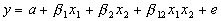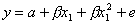Lecture 20
More on Multiple Regression

In this lecture, I would just like to discuss several miscellaneous topics related to the application of regression analysis.

On SPSS printouts, you will often see something called the "adjusted R-square." This adjusted value for R-square will be equal or smaller than the regular R-square. The adjusted R-square adjusts for a bias in R-square. R-square tends to over estimate the variance accounted for compared to an estimate that would be obtaned from the population. There are two reasons for the overestimate, a large number of predictors and a small sample size. So, with a small sample and with few predictors, adjusted R-square should be very similar the the R-square value. Researchers and statisticians differ on whether to use the adjusted R-square. I don't tend to use it if very often and I don't see it reported in the literature very often. It is probably a good idea to look at it to see how much your R-square might be inflated, especially with a small sample and many predictors.

Simultaneous vs. "Hierarchical" Regression
With any computer program, the researcher has the option of entering predictor variables into the regression analysis one at a time or in steps. For instance, one might want to run a regression analysis of the fat intake results first entering the fat intake predictor, then on the next step, entering in the dietary cholesterol intake variable. The computer then prints out the first regression equation with only fat intake as a predictor (a simple regression analysis). Then the computer re-runs the analysis with the dietary cholesterol predictor together with the fat intake variable. This allows the researcher to see the increase in the variance accounted for when the second predictor is added. SPSS prints something called the R-square change, which is just the improvement in R-square when the second predictor is added. The R-square change is tested with an F-test, which is referred to as the F-change. A significant F-change means that the variables added in that step signficantly improved the prediction.

Each stage of this analysis is usually referred to as a block. You can have as many predictor variables added in each block as you like. You should realize, however, that if you add just one predictor, the test of the F change is identical to the test of the slope of the second predictor.

If all the variables are entered into the analysis at the same time, the analysis is called a simultaneous regression. Simultaneous regression simply means that all the predictors are tested at once. I tend to use the approach most often. When the variables are entered into the equation in steps, it is sometimes referred to as "hierarchical" regression. This is a confusing term, because there is an unrelated statistical analysis called "hiearchical linear modeling" (which we are not going to cover in this class).

Exploratory Stategies
When there are many predictor variables, researchers sometimes use an exploratory analysis to see which predictors are significant predictors so that they can keep some predictors and thowout others. The most commonly used method is called "stepwise." In stepwise regression, the computer runs many regression analyses adding and subtracting predictors that are significant. It then prints a final equation with the predictors that were significant. This is generally considered a very exploratory approach and is often criticized. It turns out that this approach is not very good at coming up with the best set of predictors. Usually the goal is to find a combination of predictors that will account for the maximum amount of variance in the dependent variable.

If this is the goal, there is another option (although last time I checked, SPSS did not offer the option), called "all-subsets regression." With all-subsets regression, the computer runs all possible combinations of variables and prints it out along with some statistics that help determine which set of predictors is the best. If you are going completely exploratory in your analysis, this is the best approach.

Other options include forward stepwise and backward stepwise, in which the predictors are added one at a time or subtracted from the full model one at a time. These procedures perform even more poorly than stepwise when attempting to locate the best combination of predictors.

My editorial comment is to discourage the exploratory approach in general. I believe it is generally better to have analyses guided by theory. When there are just a few predictors, there really is no need to look for the best combination. If a researcher is really compelled to use an exploratory approach, it is probably best to go all the way by using the all-subsets method.

Dichotomous Predictors (Dummy Coding)
Dichotomous predictors can be used in multiple regression. They are typically coded as 0's and 1's, and this is referred to as dummy coding. When there are three levels of a nominal predictor variable, the researcher can dummy code the three-level variable into two new variables that allow for contrasts between combinations of two levels at a time. Daniel provides a brief discussion of this, and more can be learned from any text on multiple regression.

Interactions and Curvilinear Effects
A statistical interaction can be tested with multiple regression. Remember that I said that, mathematically speaking, interactions are multiplicative effects. To test an interaction between two predictors (independent variables), one computes a new variable that is a product of the two predictors. X1 and X2 are multiplied together. Then all three, x1, x2, and the new multiplicative term, x1x2, are entered into the analysis. This is the equation for testing an interaction effect:Of course, either of the two predictors involved (or both) can be dichotomous. When they are both dichotomous, the analysis is equivalent to factorial ANOVA. Again, to make it exactly equal an ANOVA a special coding system must be used (-1,+1), which is referred to as "effects coding."

Curvilinear effects can also be tested with multiple regression by computing a squared term. The following equation describes a test of one predictor and its squared conterpart. The first slope represents the linear relationship between x and y and the second term represents the curvilinear relationship between x and y.Mulicollinearity
When two or more predictors are highly correlated with one another, a problem can arise when conducting a regression anlaysis. This is referred to as multicollinearity. When multicollinearity is a problem, the standard errors associated with the slopes may become erroneously enormous (highly inflated). This leads to incorrect conclusions from the significance tests of the slopes. Usually, predictors can be correlated with one another as much as .8 or so before there is a problem. There are some special diagnostic tests that can be requested if there is a concern about multicollinearity. I won't go into the details here, however. One simple solutions is to drop one of the variables. When the predictors are highly correlated, it usually indicates they are measures of the same thing. So, a simple solution is to eliminate one of the redundant predictors.

The General Linear Model
Finally, I've noted several times that ANOVA is a special case of regression analysis. Regression is a more general statistical procedure and encompasses ANOVA. Any hypothesis that can be tested with ANOVA can be tested with regression. Regression is more flexible, because continuous or dichotomous predictors can be used. Because regression is concerned with the linear relationship between x and y ("linear" referring the regression line), both ANOVA and regression are considered part of the General Linear Model.